$$\def\loading{......LOADING......Please Wait} \def\RR{\bf R} \def\real{\mathbb{R}} \def\bold#1{\bf #1} \def\d{\mbox{Cord}} \def\hd{\widehat \mbox{Cord}} \DeclareMathOperator{\cov}{cov} \DeclareMathOperator{\var}{var} \DeclareMathOperator{\cor}{cor} \newcommand{\ac}{\left\{#1\right\}} \DeclareMathOperator{\Ex}{\mathbb{E}} \DeclareMathOperator{\diag}{diag} \newcommand{\bm}{\boldsymbol{#1}} \def\wait{......LOADING......Please Wait}$$

## Granger Mediation Analysis for Multiple Time Series

### Xi (Rossi) LUO

Brown University
Department of Biostatistics
Center for Statistical Sciences
Computation in Brain and Mind
Brown Institute for Brain Science
Brown Data Science Initiative
ABCD Research Group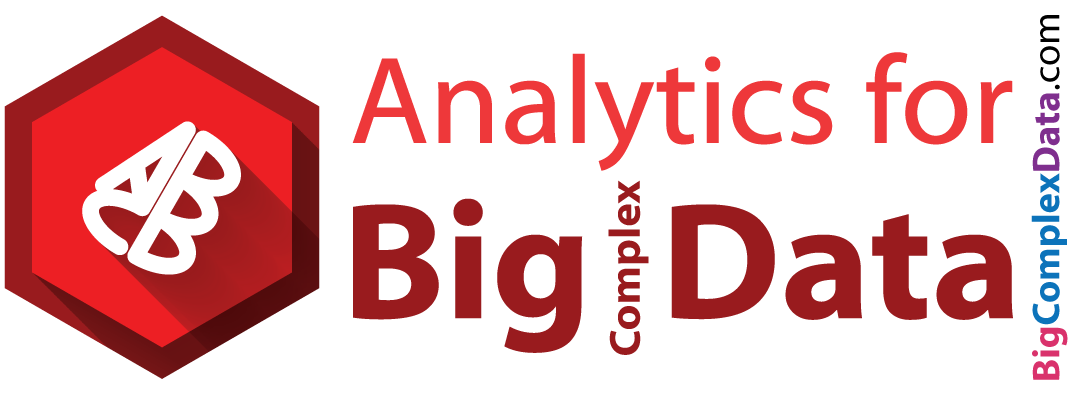ICSA, Qingdao China
July 4, 2018

Funding: NIH R01EB022911, P20GM103645, P01AA019072, P30AI042853; NSF/DMS (BD2K) 1557467

# Slides viewable on web: bit.ly/icsa18

## Co-AuthorYi Zhao
Now at Johns Hopkins Biostat

## fMRI Experiments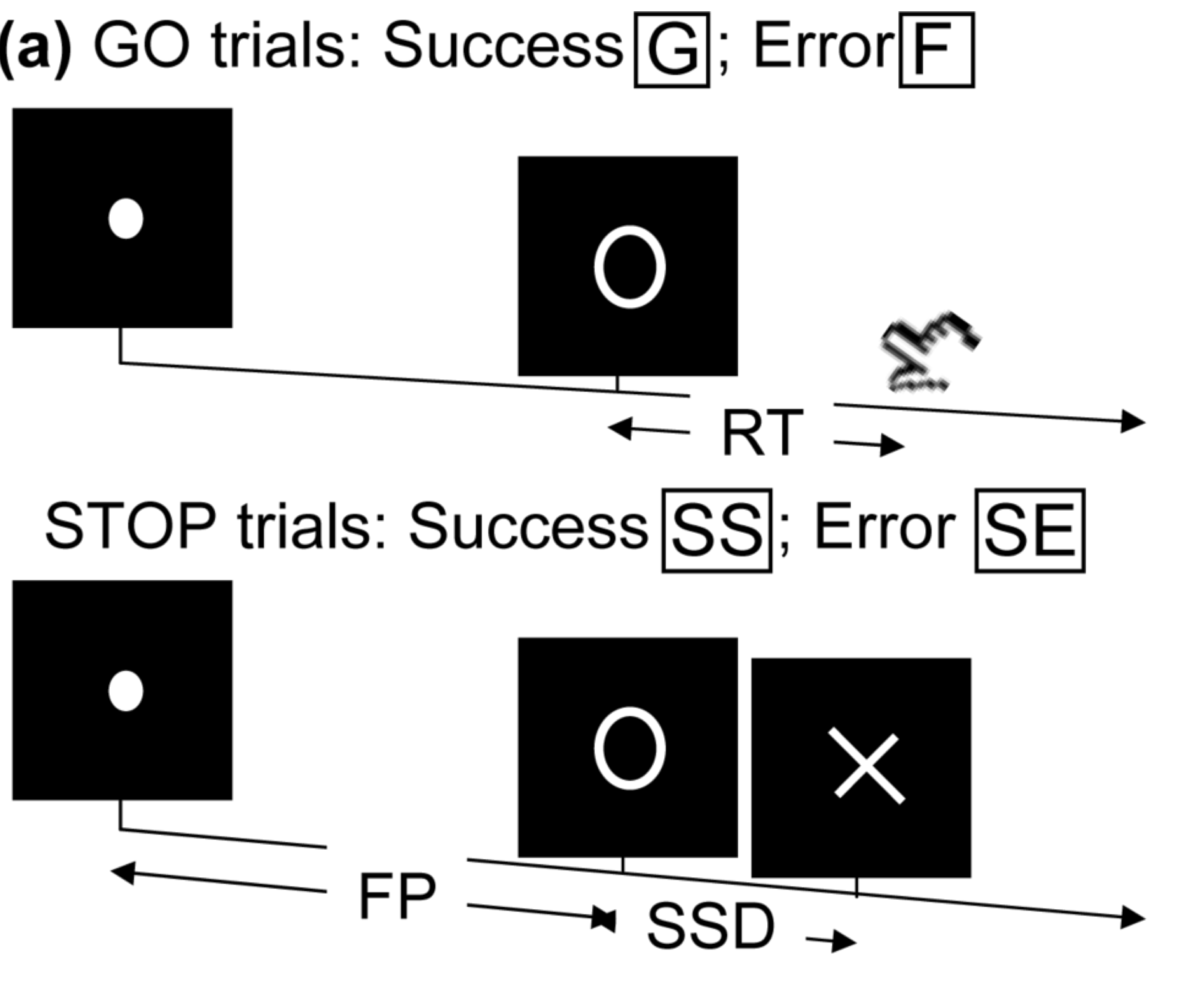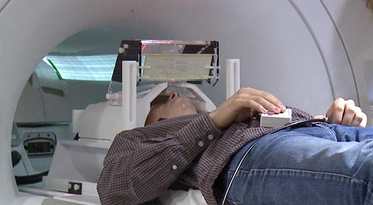• Task fMRI: performs tasks under brain scanning
• Randomized stop/go task:
• press button if "go";
• withhold pressing if "stop"
• Not resting-state: "do nothing" during scanning

Goal: infer brain activation and connectivity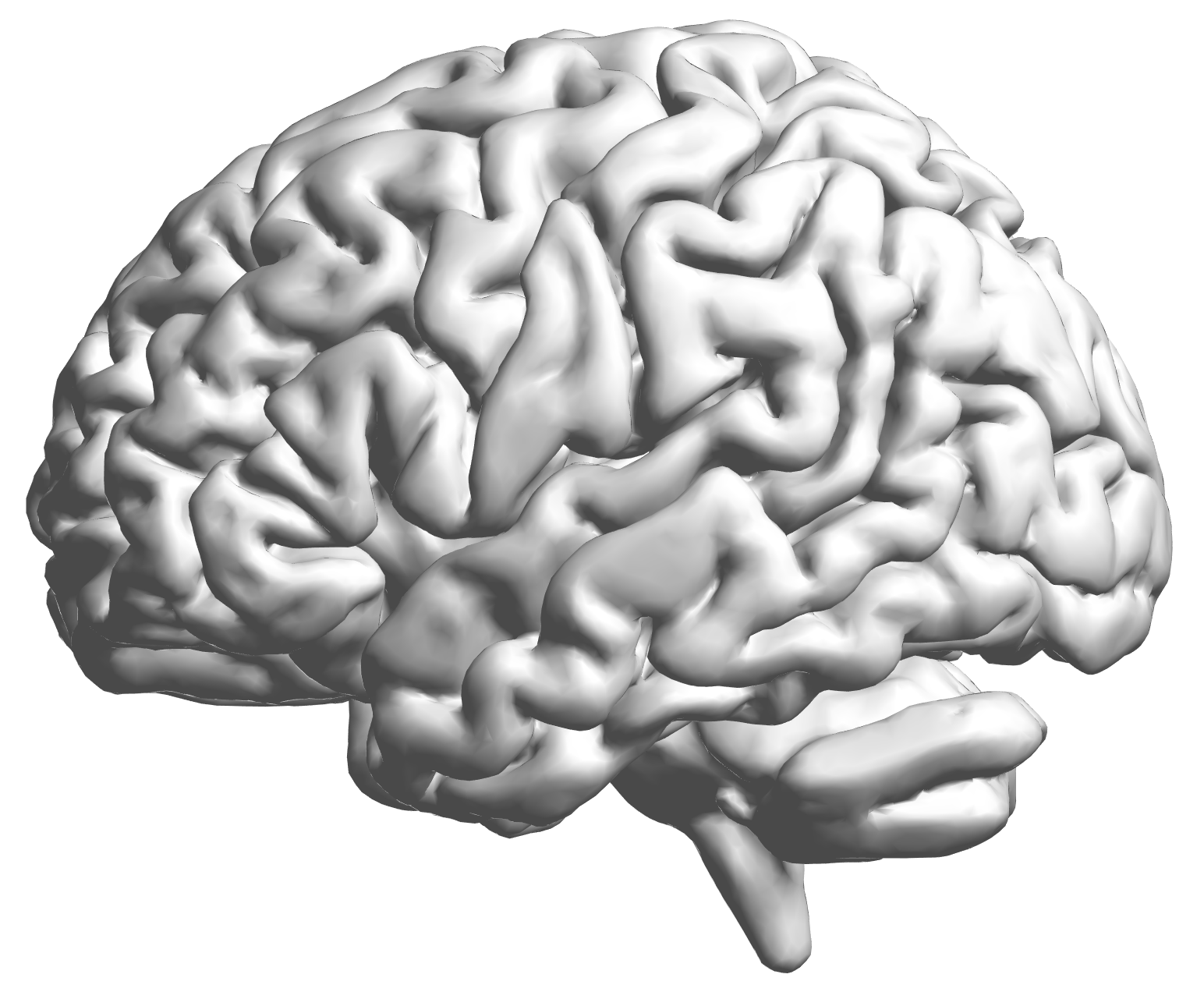fMRI data: blood-oxygen-level dependent (BOLD) signals from each cube/voxel (~millimeters), $10^5$ ~ $10^6$ voxels in total.

## Multilevel fMRI Studies

Sub 1, Sess 1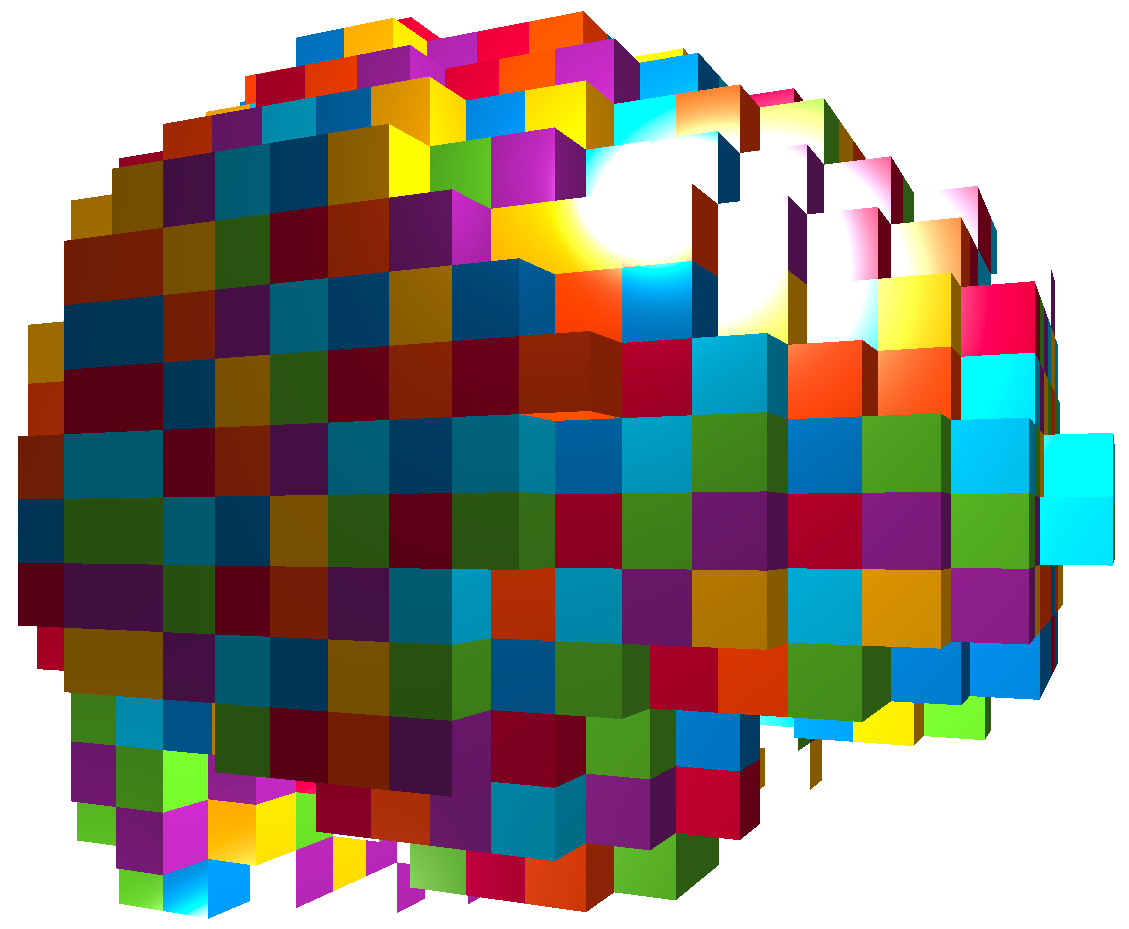Time 12~200

Sub i, Sess jSub ~100, Sess ~4Large, multilevel (subject, session, voxel) data
e.g. $1000 \times 4 \times 300 \times 10^6 \approx 1$ trillion data points

## Raw Data: Motor Region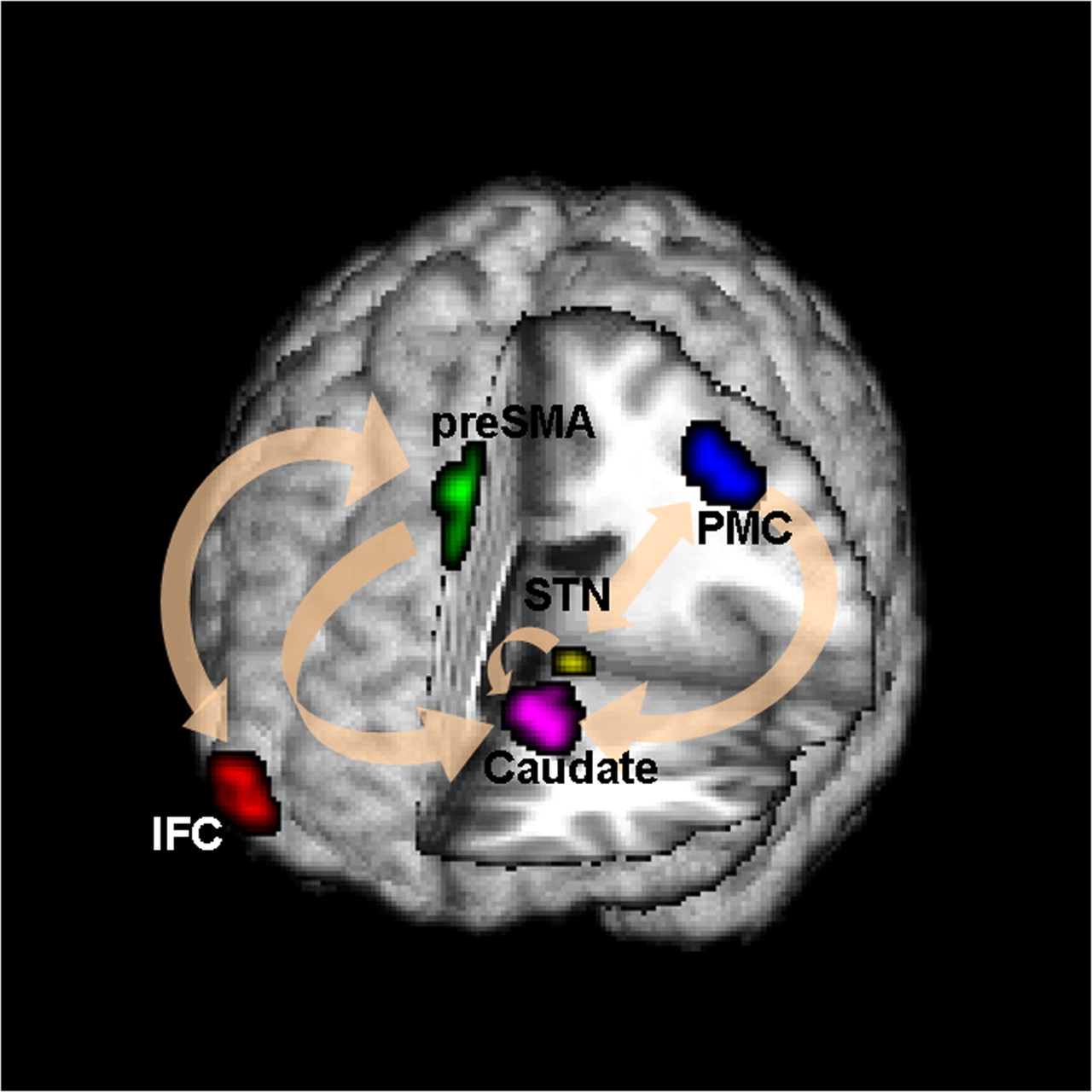Time (seconds)

Black: fMRI BOLD activity
Blue: stop onset times; Red: go onset times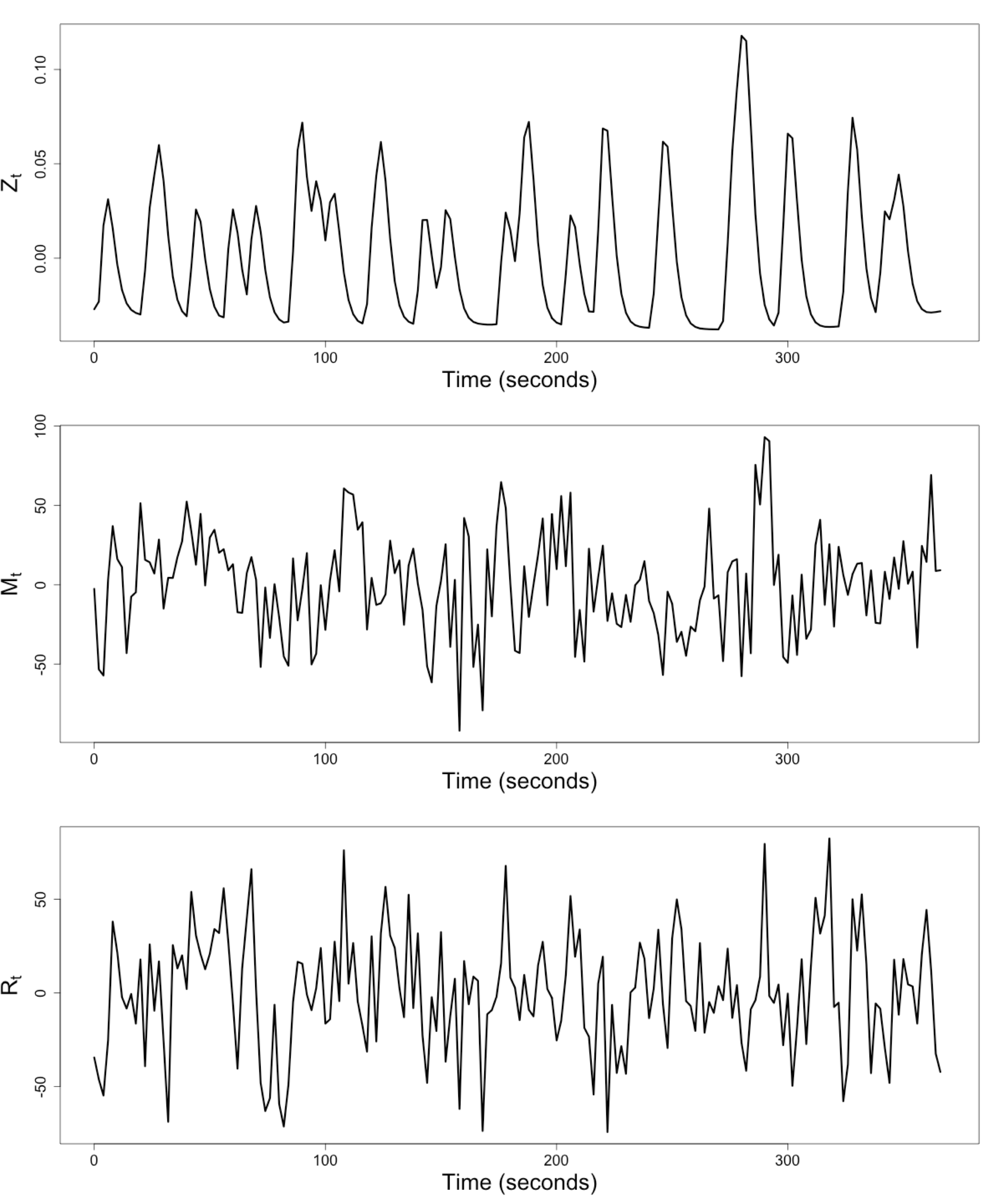$Z_t$: Stimulus onsets convoluted with Canonical HRF

$M_t$, $R_t$: fMRI time series from two brain regions

## Review: Granger Causality/VAR

• Given two (or more) time series $x_t$ and $y_t$ \begin{align*} x_t &= \sum_{j=1}^p \psi_{1j} x_{t-j} + \sum_{j=1}^p \phi_{1j} y_{t-j} + \epsilon_{1t} \\ y_t &= \sum_{j=1}^p \psi_{2j} y_{t-j} + \sum_{j=1}^p \phi_{2j} x_{t-j} + \epsilon_{2t} \end{align*}
• Also called vector autoregressive models
• $y$ Granger causes $x$ if $\phi_{1j} \ne 0$ Granger, 69
• Models pair-wise connections not pathways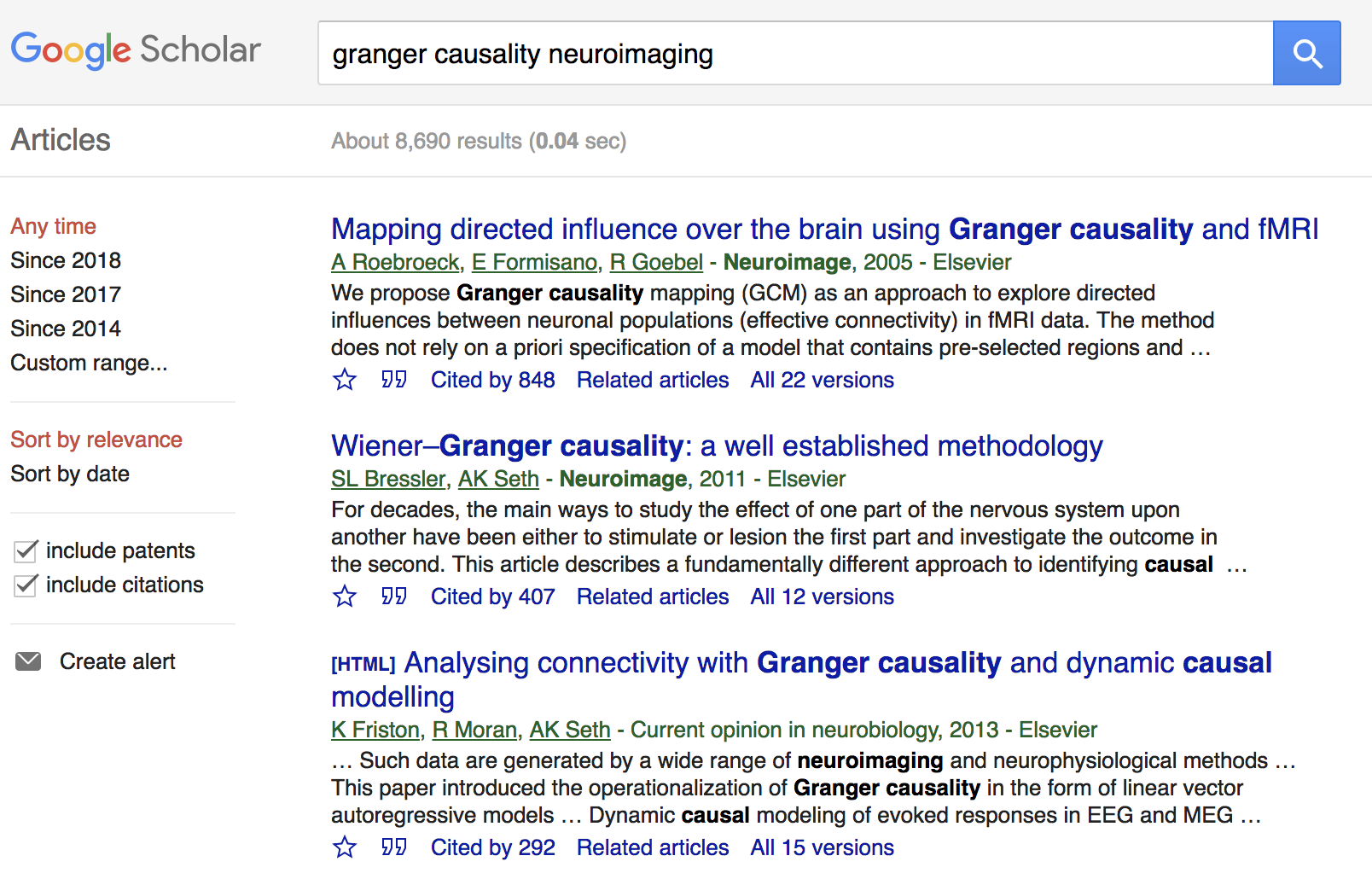## Granger Causality/VAR

• Granger Causality (VAR) popular for fMRI
• About 8000 google scholar results on "granger causality neuroimaging"
• Models multiple stationary time series
• AR($p$) (small $p$) fits fMRI well Lingdquist, 08
• Not for non-stationary/task fMRI
• Cannot model stimulus effects

## Conceptual Model with StimulusGoal: quantify effects stimulipreSMAPMC regions Duann, Ide, Luo, Li (2009). J of Neurosci

## Model: Mediation Analysis and SEM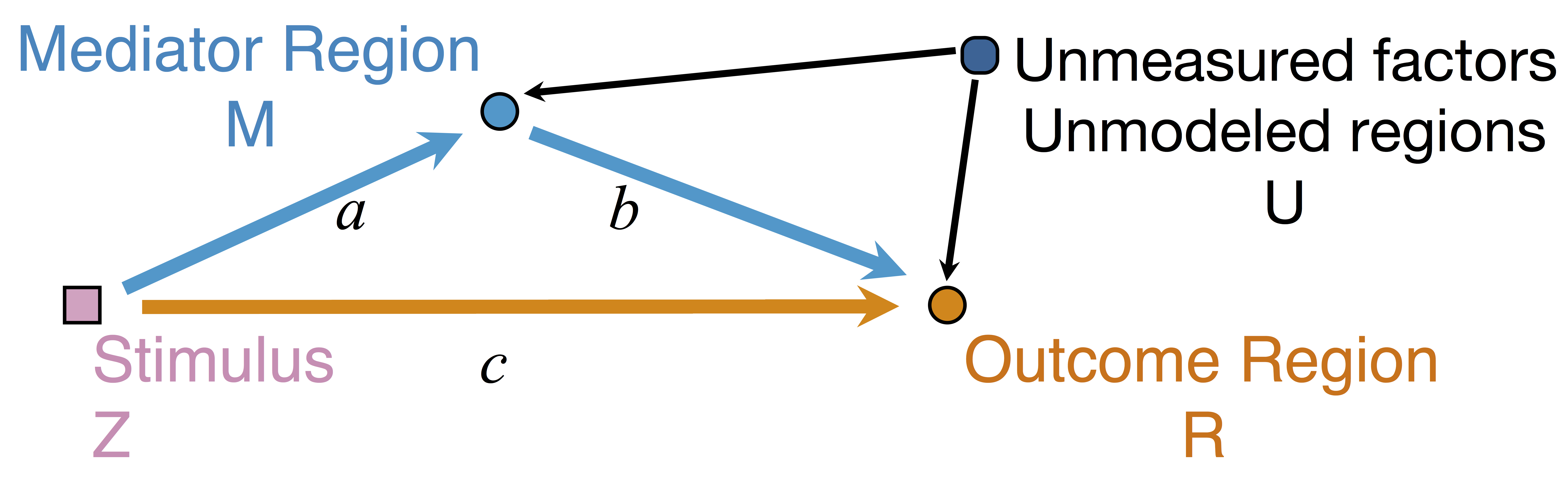\begin{align*}M &= Z a + \overbrace{U + \epsilon_1}^{E_1}\\ R &= Z c + M b + \underbrace{U g + \epsilon_2}_{E_2}, \quad \epsilon_1 \bot \epsilon_2\end{align*}
• Indirect effect: $a \times b$; Direct effect: $c$
• Correlated errors: $\delta = \cor(E_1, E_2) \ne 0$ if $U\ne 0$

## Mediation Analysis in fMRI

• Mediation analysis (usually assuming $U=0$)Baron&anp;Kenny, 86; Sobel, 82; Holland 88; Preacher&Hayes 08; Imai et al, 10; VanderWeele, 15;...
• Parametric Wager et al, 09 and functional Lindquist, 12 mediation, under (approx.) independent errors
• Stimulus $\rightarrow$ brain $\rightarrow$ user reported ratings, one brain mediator
• Assuming $U=0$ between ratings and brain
• Multiple mediator and multiple pathways
• Dimension reduction by arXiv1511.09354Chen, Crainiceanu, Ogburn, Caffo, Wager, Lindquist, 15
• Pathway Lasso penalization Zhao, Luo, 16
• This talk: integrating Granger causality and mediation analysis

# Model & Method

## Our Mediation Model

\begin{align*}M_{t} = Z_{t} a + E_{1t},\quad R_t = Z_t c + M_t b + E_{2t}\end{align*}
• Temporal VAR errors \begin{align*} E_{1t}&=& \sum_{j=1}^{p}\left(\omega_{11_{j}}E_{1,t-j}+\omega_{21_{j}}E_{2,t-j}\right)+\epsilon_{1t} \\ E_{2t}&=&\sum_{j=1}^{p}\left(\omega_{12_{j}}E_{1,t-j}+\omega_{22_{j}}E_{2,t-j}\right)+\epsilon_{2t} \end{align*}
• Spatial errors: $\epsilon_{1t}, \epsilon_{2t}$ $$\begin{pmatrix} \epsilon_{1t} \\ \epsilon_{2t} \end{pmatrix}\sim\mathcal{N}\left(\boldsymbol{\mathrm{0}},\boldsymbol{\Sigma}\right), \quad \boldsymbol{\Sigma}=\begin{pmatrix} \sigma_{1}^{2} & \delta\sigma_{1}\sigma_{2} \\ \delta\sigma_{1}\sigma_{2} & \sigma_{2}^{2} \end{pmatrix}$$

## Equivalent Form

\begin{align*} \scriptsize M_{t}& \scriptsize =Z_{t}A+\sum_{j=1}^{p}\left(\phi_{1j}Z_{t-j}+\psi_{11_{j}}M_{t-j}+\psi_{21_{j}}R_{t-j}\right)+\epsilon_{1t} \\ \scriptsize R_{t}& \scriptsize =Z_{t}C+M_{t}B+\sum_{j=1}^{p}\left(\phi_{2j}Z_{t-j}+\psi_{12_{j}}M_{t-j}+\psi_{22_{j}}R_{t-j}\right)+\epsilon_{2t} \end{align*}
• Nonzero $\phi$'s and $\psi$'s denote the temporal influence from stimulus to mediator/outcome and etc
• $A$, $B$, $C$ are causal following a similar proof in Sobel, Lindquist, 04

## Estimation: Conditional Likelihood

• The full likelihood for our model is too complex
• Given the initial $p$ time points, the conditional likelihood is \begin{align*} & \tiny \ell\left(\boldsymbol{\Theta},\delta~|~\mathbf{Z},\mathcal{I}_{p}\right) = \sum_{t=p+1}^{T}\log f\left((M_{t},R_{t})~|~\mathbf{X}_{t}\right) \\ & \tiny = -\frac{T-p}{2}\log\sigma_{1}^{2}\sigma_{2}^{2}(1-\delta^{2})-\frac{1}{2\sigma_{1}^{2}}\|\mathbf{M}-\mathbf{X}\boldsymbol{\theta}_{1}\|_{2}^{2} \\ & \tiny -\frac{1}{2\sigma_{2}^{2}(1-\delta^{2})}\|(\mathbf{R}-\mathbf{M}B-\mathbf{X}\boldsymbol{\theta}_{2})-\kappa(\mathbf{M}-\mathbf{X}\boldsymbol{\theta}_{1})\|_{2}^{2} \end{align*}

## Multilevel Data: Two-level Likelihood

• Second level model, for each subject $i$ $$(A_i,B_i,C_i) = (A,B,C) + (\eta^A_i, \eta^B_i, \eta^C_i)$$ where errors $\eta$ are normally distributed
• The two level likelihood is conditional convex
• Two-stage fitting: plug-in estimates from the first level
• Block coordinate fitting: jointly optimize first level likelihood + second level likelihood
Theorem: Assume assumptions (A1)-(A6) are satisfied. Assume $\mathbb{E}(Z_{i_{t}}^{2})=q\lt \infty$, for $i=1,\dots,N$. Let $T=\min_{i}T_{i}$.
1. If $\boldsymbol{\Lambda}$ is known, then the two-stage estimator $\hat{\delta}$ maximizes the profile likelihood of model asymptotically, and $\hat{\delta}$ is $\sqrt{NT}$-consistent.
2. If $\boldsymbol{\Lambda}$ is unknown, then the profile likelihood of model has a unique maximizer $\hat{\delta}$ asymptotically, and $\hat{\delta}$ is $\sqrt{NT}$-consistent, provided that $1/\varpi=\bar{\kappa}^{2}/\varrho^{2}=\mathcal{O}_{p}(1/\sqrt{NT})$, $\kappa_{i}=\sigma_{i_{2}}/\sigma_{i_{1}}$, $\bar{\kappa}=(1/N)\sum\kappa_{i}$, and $\varrho^{2}=(1/N)\sum(\kappa_{i}-\bar{\kappa})^{2}$.
Using the two-stage estimator $\hat{\delta}$, the CMLE of our model is consistent, as well as the estimator for $\mathbf{b}=(A,B,C)$.

## Theory: Summary

• Under regularity conditions, $N$ subs, $T$ time points
• Our $\hat \delta$ is $\sqrt{NT}$-consistent
• This relaxes the unmeasured confounding assumption in mediation analysis
• Our $(\hat{A},\hat{B}, \hat{C})$ is also consistent

# Simulations & Real Data

## Comparison

• Our methods: GMA-h and GMA-ts
• Previous methods: BK Baron & Kenny, MACC Zhao and Luo, KKB Kenny et al
• Other methods do not model the temporal correlations or time series like ours

## SimulationsLow bias for $AB$Low bias for temporal cor

Gray dash lines are the truth

GMA performs the best, and recovers the temporal correlations

## Real Data Experiment

• Public data: OpenFMRI ds30
• Stop-go experiment: withhold (STOP) from pressing buttons
• Expect "STOP" stimuli to deactivate brain region M1
• Goal: quantify the role of region preSMA

## Result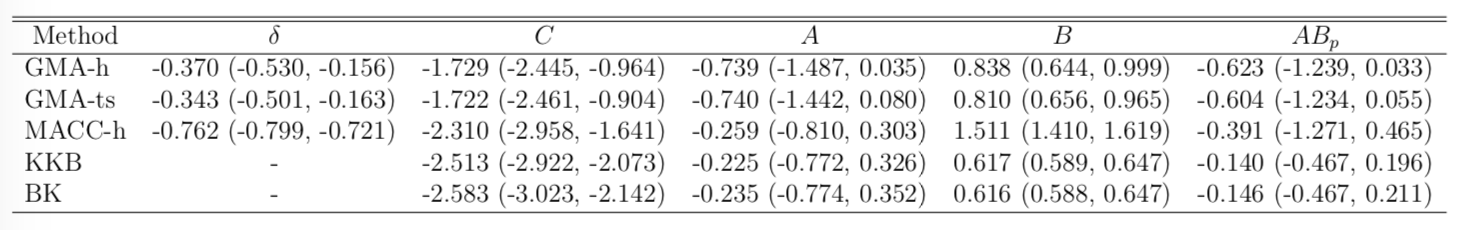• STOP deactivates M1 directly ($C$) and indirectly ($AB$)
• preSMA mediates a good portion of the total effect
• Help resolve the debates among neuroscientists
• Other methods under-estiamte the effects
• Novel feedback findings: M1 → preSMA after lag 1 and 2 (not shown)

## Discussion

• Mediation analysis for multiple time series
• Method: Granger causality + mediation
• Optimizing complex likelihood
• Theory: identifiability, consistency
• Result: low bias and improved accuracy
• Extension: functional mediation (Zhao, Function 37)
• CRAN pkg: gma and references within